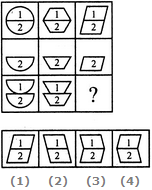# Non Verbal Reasoning - Figure Matrix - Discussion

Discussion Forum : Figure Matrix - Section 1 (Q.No. 4)
Directions to Solve

In each of the following questions, find out which of the answer figures (1), (2), (3) and (4) completes the figure matrix ?

4.

Select a suitable figure from the four alternatives that would complete the figure matrix.1
2
3
4
Explanation:
In each column, the second figure (middle figure) is obtained by removing the upper part of the first figure (uppermost figure) and the third figure (lowermost figure) is obtained by vertically inverting the upper part of the first figure.
Discussion:
21 comments Page 1 of 3.

Geethu said:   2 years ago
I think the answer is A.
(1)

Moon said:   4 years ago
Answer c is right. If you keep the reflection of the upper part of parallelogram and imagine the coordinates across which the reflection occurs and fix the lower part, you will get the figure C.
(1)

Sathik said:   5 years ago
A is the correct answer. Here figure 2 inverted image looks like figure 1.
(1)

Rishi Tandon said:   6 years ago
In my view, A is the correct answer.
(1)

Ishika said:   6 years ago
Why not D?
(1)

Yogi said:   7 years ago

The 1st half of the series in the 1st figure is in opposite direction for the 1st half of 3rd figure.

Avve said:   7 years ago
@Dr. Helen Ravi Martha.

It couldn't be the 4. There isn't any hint suggesting that the shapes have been flipped.

I think that the right ones are the 1 and the 3. But I can't understand what are the number for Tthe puzzle would have worked without them in the very same (bad) way.

Or maybe the numbers are the key for preferring the third option over the first. But I don't see the point. If there was a rotation of the upper shape, the number 1 should be rotated to, while if there was a horizontal reflection, also the 1 should be reflected. But the image hasn't enought resolution to exclude that in the original pattern (printed somewhere) the 1 is flipped horizontally so that its "head" is upside down.

Vivek said:   7 years ago
I think, option A is the right answer.
(1)

Dr .helen Ravi Martha said:   7 years ago
Ok all of you are wrong.

The rite answer is 4. But 3 can be correct too.

Based on my deduction as answer 4 is similar to answer 3 (given answer) just flipped the opposite direction and those in column 1 and 2 cannot be confirmed if it is flipped or the same direction.

If all of you do not agree you can all go and look.

But the conclusion is, this question has many answers.

Hope that helped.

Anu said:   8 years ago
A is also correct. With different logic you can choose either A or C.